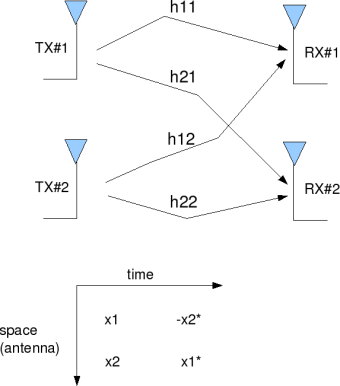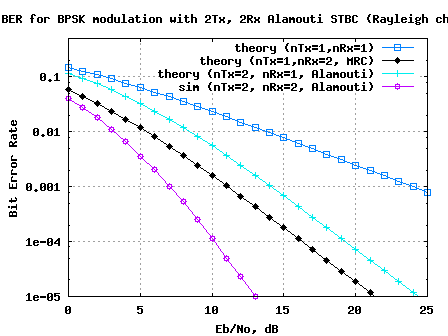- DSP log - http://www.dsplog.com -

Alamouti STBC with 2 receive antenna

Posted By Krishna Sankar On March 15, 2009 @ 9:01 pm In MIMO | 147 Comments

In the past, we had discussed two transmit, one receive antenna Alamouti Space Time Block Coding (STBC)  scheme. In this post, lets us discuss the impact of having two antennas at the receiver. For the discussion, we will assume that the channel is a flat fading Rayleigh multipath channel  and the modulation is BPSK.

## Alamouti STBC with two receive antenna

The principle of space time block coding with 2 transmit antenna and one receive antenna is explained in the post on Alamouti STBC . With two receive antenna’s the system can be modeled as shown in the figure below.Figure: 2 Transmit 2 Receive Alamouti STBC

For discussion on the channel and noise model, please refer to the post on two transmit, one receive antenna Alamouti Space Time Block Coding (STBC)  scheme.

The received signal in the first time slot is,

.

Assuming that the channel remains constant for the second time slot, the received signal is in the second time slot is,

where

are the received information at time slot 1 on receive antenna 1, 2 respectively,

are the received information at time slot 2 on receive antenna 1, 2 respectively,

is the channel from receive antenna to transmit antenna,

, are the transmitted symbols,

are the noise at time slot 1 on receive antenna 1, 2 respectively and

are the noise at time slot 2 on receive antenna 1, 2 respectively.

Combining the equations at time slot 1 and 2,

.

Let us define .

To solve for , we know that we need to find the inverse of .

We know, for a general m x n matrix, the pseudo inverse  is defined as,

.

The term,

Since this is a diagonal matrix, the inverse is just the inverse of the diagonal elements, i.e

The estimate of the transmitted symbol is,

.

## Simulation Model

The Matlab/Octave script performs the following

(a) Generate random binary sequence of +1′s and -1′s.

(b) Group them into pair of two symbols

(c) Code it per the Alamouti Space Time code, multiply the symbols with the channel and then add white Gaussian noise.

(e) Perform hard decision decoding and count the bit errors

(f) Repeat for multiple values of and plot the simulation and theoretical results.Figure: BER plot for 2 transmi 2 receive Alamouti STBC

## Observations

1. Can observe that the BER performance is much better than 1 transmit 2 receive MRC case. This is because the effective channel concatenating the information from 2 receive antennas over two symbols results in a diversity order of 4.

2. In general, with receive antennas, the diversity order for 2 transmit antenna Alamouti STBC is .

3. As with the case of 2 transmit, 1 receive Alamouti STBC , the fact that is a diagonal matrix ensured that there is no cross talk between , after the equalizer and the noise term is still white.

## Reference

[DIG-COMM-BARRY-LEE-MESSERSCHMITT] Digital Communication: Third Edition, by John R. Barry, Edward A. Lee, David G. MesserschmittA  Simple Transmit Diversity Technique for Wireless Communication Siavash M Alamouti, IEEE Journal on selected areas in Communication, Vol 16, No, 8, October 1998

URLs in this post:

 two transmit, one receive antenna Alamouti Space Time Block Coding (STBC): http://www.dsplog.com/2008/10/16/alamouti-stbc/

 Rayleigh multipath channel: http://www.dsplog.com/2008/07/14/rayleigh-multipath-channel/

 pseudo inverse: http://planetmath.org/encyclopedia/Pseudoinverse.html

 Matlab/Octave script for computing BER for 2 transmit 2 receive Alamouti STBC for BPSK modulation in flat fading Rayleigh channel: http://www.dsplog.com/db-install/wp-content/uploads/2009/03/script_ber_2x2_alamouti_stbc_code_bpsk_rayleigh_channel.m